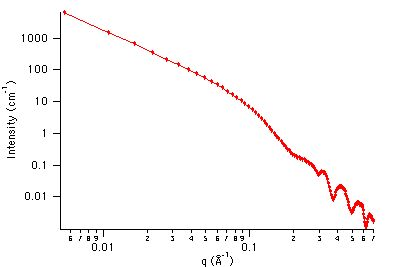MODEL

Ellipsoid

AUTHOR/MODIFICATION

Steve Kline 20 JAN 1999

Alan Munter 08 JULY 1999, converted to Java

APPROVED FOR DISTRIBUTION

DESCRIPTION

Calculates the form factor for a monodisperse ellipsoid (ellipsoid of revolution) with uniform scattering length density. The form factor is normalized by the particle volume such that P(q) = scale*<f*f>/Vol + bkg, where f is the scattering amplitude and the < > denote an average over all possible orientations of the ellipsoid.

Resolution smeared version is also provided.

VARIABLES

Input Variables (default values):

Parameter Variable Value
0Scale1.0
1Ra (rotation axis) (Å)20.0
2Rb (Å)400.0
3Contrast (Å-2)3.0e-6
4Incoherent Background (cm-1)0.0

USAGE NOTES

The function calculated is: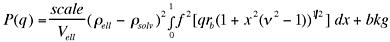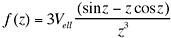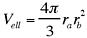and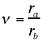The returned value is scaled to units of [cm-1], on absolute scale.

The ellipse is rotated about the axis of Ra to define the ellipsoid. If (as shown below) the Rb >Ra, the object is an oblate ellipsoid (disk-like). If Rb < Ra, then the object is a prolate ellipsoid (needle-like). If the two radii are equal, then the ellipsoid is a sphere.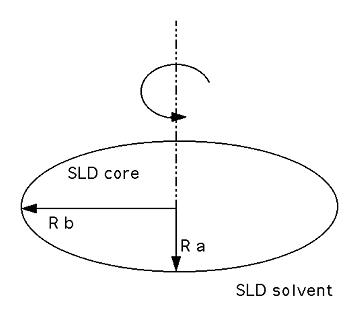If the object is an oblate ellipsoid (disk-like), it displays a limiting power-law slope of q-2 at low q.

A prolate ellipsoid (needle-like), displays a limiting power-law slope of q-1 at low q.

Parameter (scale) and Parameter (contrast) are both multiplicative factors in the model and are perfectly correlated. One or both of these parameters must be held fixed during model fitting.

If the scale factor Parameter is set equal to the particle volume fraction, phi, the returned value is the scattered intensity per unit volume, I(q) = phi*P(q). However, no interparticle interference effects are included in this calculation.

REFERENCE

Feigin, L. A, and D. I. Svergun, "Structure Analysis by Small-Angle X-Ray and Neutron Scattering", Plenum Press, New York, (1987).

TEST DATASET

This example dataset is produced by calculating the Ellipsoid using 128 data points, qmin = 0.001 Å-1, qmax = 0.7 Å-1 and the above default parameter values.